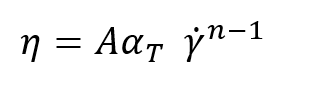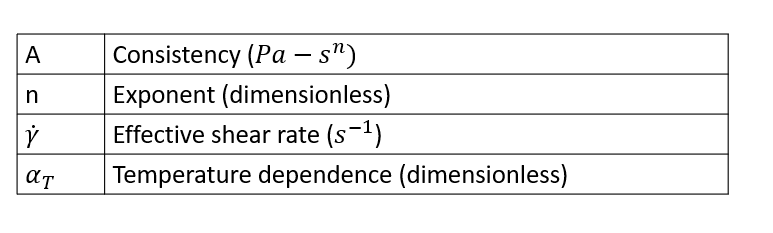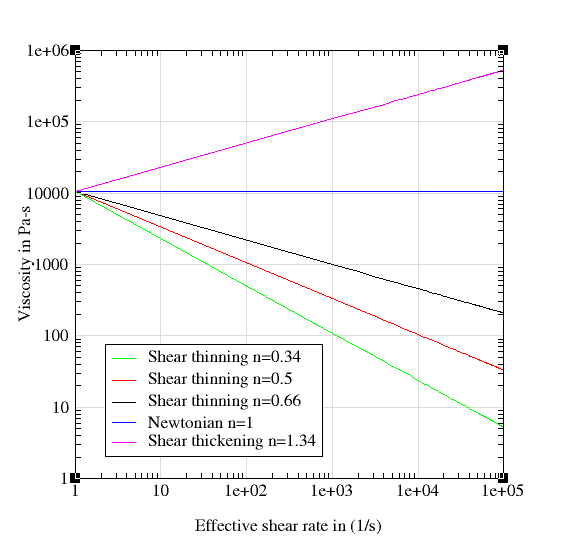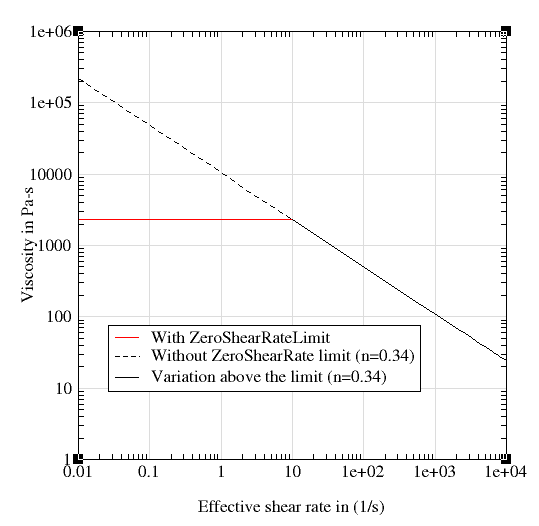# Power Law Model

This is a two parameter model and it is described by the following equation.This model describes the viscosity using a power-law relationship. The exponent n determines the nature of the relationship.

n < 1 Shear thinning or Pseudoplastic

n > 1 Shear thickening or dilalatant

n = 1 Newtonian

For most polymers, Exponent n is less than 1. When the exponent is less than 1, viscosity of the polymer decreases with increase in the shear rate. This behavior is called shear thinning. On the other hand, when the exponent is greater than 1, viscosity increases with shear rate and this behavior is called shear thickening. The following figure shows viscosity as a function of exponent (consistency = 1.05e+04)One of the disadvantages of the power-law model is, as the shear rate tends to zero (when the exponent is less than 1) the viscosity tends to infinity. This setback is overcome in HyperXtrude by defining a ZeroShearRateLimit. Typically, this value is chosen based on the experimental data used to determine the model coefficients. For instance, if the ZeroShearRateLimit is set to 10.0, then HyperXtrude will use this value to compute the viscosity whenever the effective shear rate of the flow field is less than the ZeroShearRateLimit. The following figure shows this effect for a power-law model with consistency 1.05e+04 and exponent 0.34.In addition to this, a few additional parameters arise due tot he temeprature dependence of material data; these variables are discussed in Temperature Dependence.

## Syntax

 Polymer PolymerName { ConstituveModel = "PowerLaw" Density = ρ SpecificHeat = Cp (T) Conductivity = K (T) CoeffOfThermalExpansion = βT VolumetricHEatSource = Qvol Consistency = A Exponent = n ZeroShearRate = γ0 TemperatureDependence = "None" }

## Explanation of Parameters

Parameter Description Units Data Type Condition Typical Value
ConstitutiveModel Describes the model used None String Required "PowerLaw"
Density Density fo the polymer kg/m^3 Constant Required 995.0
SpecificHeat Specific heat at constant pressure J/kg/K Constant/F(T) Required 2000.0
COnductivity Thermal conductivity W/m/K Constant/ F(T) Required 0.167
CoeffOfThermalExpansion Indicates the change in volume with change in temperature 1/K Constant Required 1.0e-05
VolumetricHeatSource Heat generated/removed in the volume by methods like electrical heating W/m^3 Constant Required 0.0
Consistency One of the parameters of the power law model. When n=1 it is the same as viscosisty. pa s^n Constant Required 1.0e+04
Exponent Power law index, defines the dependency of viscosity on shear rate. None Constant Required 0.66
ZeroShearRateLimit See above 1/s Constant Required 0.01
TemperatureDependence See Temperature Dependence None String Required "Exp(-Beta(DeltaT))"
ReferenceTemperature Temperature at which data is calculated for the intialization step. K Constant Required only if TD is not "None" 533
FreezeTemeprature This ist eh no flow temperature. Below this temperature, material ceases to flow. K Constant Required only if TD is not "none" 350
ActivationEnergy A parameter required by Arrhenius model. J/mol Constant Required only if TD is Exp(Q/RT) 16628
UniversalGasConstant A parameter from state equation PV=nRT, R is universal gas constant. J/mol/K Constant Required only if TD is Exp (Q/RT) 8.314
TemperatureSensitivity A derived parameter which has the same physical meaning as Q/R. K Constant Required only if TD is Exp(Tb/T) 2000 K
WLFConstant 1 Constant C1 of WLF model None Constant Required only if TD is WLF 17.44
WLFConstant2 Constant C2 of WLF model. This is like DeltaT, hence the value is same in K and Celsius. K Constant Required only if TD is WLF 51.6
GlassTransitionTemperature Temperature below with polymer molecules ceases to move (frozen). There are few definitions of this term K Constant Required only if TD is WLF 320
Beta Parameter in the relationship Exp(-BetaDeltaT)) None Constant Required only if TD is Exp(-Beta(DeltaT)) 0.005

F(T) - Funciton of Temperature. Can be specified as a TABLE1 or TCL function.

TD - TemperatureDependence.